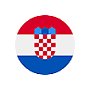# Source code of 6.1.5 - Sorting by name length```10 CLS ' Clear the screen 11 CLEAR ' Delete the contents of all variables 12 DEFINT B, I-K, M-N 13 DEFLNG D-G 16 DEFSTR P-S 20 REM Than this number further starts the program code 30 DIM A\$(9): DIM B(9) 40 N = 0 50 REM Place to return by choice 100 PRINT "Enter a name in " N; ". the matrix" : INPUT A\$(N) 110 B(N) = LEN(A\$(N)) 120 N = N + 1 130 IF N <= 9 THEN GOTO 50 200 GOSUB 1100 210 N = 0 FOR N = 0 TO 9 PRINT N, A\$(N) PRINT NEXT N 900 SLEEP 999 END ' Completion of the programming code 1000 REM Than this number further start sub-programs 1100 I = 0: J = 0: N = 9 1200 REM Place to return by choice IF B(I) < B(J) THEN GOTO 1400 ELSE nohigher = B(I): nodata\$ = A\$(I) B(I) = B(J): A\$(I) = A\$(J) B(J) = nohigher: A\$(J) = nodata\$ END IF 1400 IF J < N THEN J = J + 1: GOTO 1200 1600 IF I < N THEN I = I + 1: J = I: GOTO 1200 2000 RETURN```

In line 200 of the program is a call for the sub-program. Sub-programs always end with a RETURN command, to resume execution of the main program of the next line after the call sub-program, in this case from the line of 210.

If there is a need in a computer program to calculate several dozen times the same function, but each time with a different input variables, mathematical apparatus of functions in the program is well to set in the sub-program. Why?

It should be noted that it is not necessary to write the numbers of individual lines, as in the example shown in the display FOR-NEXT and IF-THEN-ELSE-END IF programming structures. How, then, the program jump on command GOTO? Read the Help pages (RTFM)!Citing of this page: Radic, Drago. " IT - Informatics Alphabet " Split-Croatia. {Date of access}. . Copyright © by Drago Radic. All rights reserved. | Disclaimer